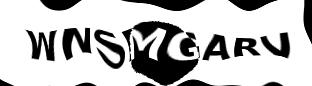Euler's Tonnetz is a scheme for arraying tones:

```   G+  D+  A+  F   C   G   D   A   E   B   F+  C+

C   G   D   A   E   B   F+  C+  G+  D+  A+  F

E   B   F+  C+  G+  D+  A+  F   C   G   D   A

G+  D+  A+  F   C   G   D   A   E   B   F+  C+

C   G   D   A   E   B   F+  C+  G+  D+  A+  F

E   B   F+  C+  G+  D+  A+  F   C   G   D   A

G+  D+  A+  F   C   G   D   A   E   B   F+  C+

C   G   D   A   E   B   F+  C+  G+  D+  A+  F
```

A step to the right in this array corresponds to a fifth and a step downwards is a major third. Triads have a triangular shape; there are two kinds of triangles: The triangles for major triads lie above their hypotenuse and those for minor triads below their hypotenuse.

Each of these triangles shares each edge with one of three triangles of the other type. For example, you can, by changing a single tone, transition from a major triad to one of three different minor triads. So I chose an arbitrary path through the triads of the Tonnetz, marked here with digits and lowercase letters from 1 through q:

```   E   B   F+  C+  G+  D+  A+  F   C   G

G+  D+  A+  F   C   G   D   A   E   B
4 5 6 7 8 9
C   G   D   A   E   B   F+  C+  G+  D+
1 2 3           a b c d e f g h
E   B   F+  C+  G+  D+  A+  F   C   G
j i
G+  D+  A+  F   C   G   D   A   E   B
l k
C   G   D   A   E   B   F+  C+  G+  D+
n m
E   B   F+  C+  G+  D+  A+  F   C   G
q p o
G+  D+  A+  F   C   G   D   A   E   B
```
(Between
`9`
and
`a`
, a minor triad is skipped.)

Upon entering the corresponding notes into my synthesizer, I messed up the note values at some point, which gave the whole thing a more interesting rhythm. Then I worked in some adornments, and that's how it sounds:
(mp3, wav)
Once again, slower:
(mp3, wav)
This version I made after I had significantly extended the sound possibilities of my synthesizer:
(mp3, wav)

Contact and legal info | © 2016-07-31 siquod.org

## Discussion

No entries yetEnter the preceding text: